Markets
Futures
Earn
More
Cancel
FAQAccount FunctionsTutorialBinance Fan TokenBinance EarnCrypto Deposit/Withdrawal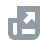Crypto Derivatives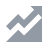FinanceAPISecurityOther Topics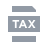Tax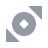Binance Convert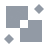NFTVIP
Home
Support Center
FAQ
Crypto Derivatives
Futures Contracts
Futures Guide
How to Visualize the Breakeven Price of Open Positions on Binance Futures (App)

# How to Visualize the Breakeven Price of Open Positions on Binance Futures (App)

2023-02-22 14:04

## What is the Breakeven Price in futures trading?

On Binance Futures, the Breakeven Price of a position is the price level at which the position would neither make nor lose profit. If the market price of a given asset is equal to the Breakeven Price of a position, you won’t experience any gains or losses.

## How to visualize the Breakeven Price for your positions in USDⓈ-M or COIN-M Futures on the Binance App?

Before you get started, you need to make sure that Detailed Mode is activated.
2. Then tap [ … ] on the top right corner and tap [Preferences] - [Positions Display Mode] and select [Detailed Mode].
Learn how to switch to Detailed Mode from Introduction to Detailed Mode and Brief Mode on Binance Futures (App).
2. On your open position, tap [Entry Price], a pop-up window displaying the Breakeven Price of your position will appear.

## How is the Breakeven Price calculated?

The Breakeven Price is calculated based on the average entry price of the whole position, including its size and direction. If a trader enters into a position at different price levels or in different quantities, the Breakeven Price is calculated as the average price of all the entries.
*trading fees and funding fees are not currently included in the calculation of the Breakeven Price on Binance and in the examples below.
To calculate the Breakeven Price, the formula is as follows:
Breakeven Price = (Total Cost of Position - Total Gained from Position) / Current Holding Position Size
Current holding position size: is the total size of the position, taking into account both the direction (long or short) and the asset quantity.
Note: the position size value can be negative if the position is short.
For long positions:
Total Cost of Position: is the sum of the cost of all the entries (long orders opened) in the position.
Total Gained from Position: is the sum of the gain from all short/partially-closed orders in the position.
For short positions:
Total Cost of Position: is the sum of the cost of all the closed short orders in the position.
Total Gained from Position:is the sum of the gain from all short orders opened in the position.
It's important to note that the Breakeven Price in futures is only a theoretical value. The actual trading results may differ due to factors such as slippage, trading fees, funding fees and market volatility.
Example of Breakeven Price calculation:
Let’s break down the calculation of the Breakeven Price of a position with multiple entries.
Assuming you opened the following three long positions on Binance Futures:
• Long BTCUSDT position opened at BTC price of 20,000 USDT, with a size of 0.5 BTC
• Long BTCUSDT position opened at BTC price of 22,000 USDT, with a size of 1.5 BTC
• Long BTCUSDT position opened at BTC price of 25,000 USDT, with a size of 0.5 BTC
The Breakeven Price for this position will be calculated as follows:
Breakeven Price = (total cost of position) / (position size) = (20,000*0.5+22,000*1.5+25,000*0.5) / (0.5+1.5+0.5) = 22,200 USDT
Now assuming the BTC price moves up to 25,000 USDT, rising above your Breakeven Price of 22,200 USDT. You decide to take some profit and partially close this BTCUSDT position at a BTC 25,000 USDT for a size of 0.5 BTC.
Your new Breakeven Price will then be calculated as:
New Breakeven Price =(20,000*0.5 + 22,000*1.5 + 25,000*0.5 - 25,000*0.5 ) / (0.5 + 1.5 + 0.5 - 0.5) = 21,500 USDT
How is the Breakeven Price useful in futures trading?